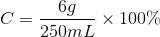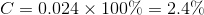# GED Science : Chemistry

## Example Questions

### Example Question #1 : Solids, Liquids, And Gases

Molecules are constantly moving. In which state of matter do molecules move the fastest?

Solids

Molecules move at the same rate in both gases and liquids

Liquids

Molecules move at the same rate in all three states of matter

Gases

Gases

Explanation:

The movement of particles is important in differentiating the states of matter. Solids have the lowest molecular energy; their molecules barely vibrate and are generally trapped in lattice structures. Molecules in liquids are able to move more freely, resulting in the fluidity of the liquid state. Gas molecules have even more freedom of movement, allowing the gas to expand to fill a space.

Consider that energy (such as heat) must be added to convert a solid to a liquid or a liquid to a gas. That energy goes to make the molecules move faster and increase the temperature of the compound. Gases require the most energy (consider boiling water), and will have the fastest moving molecules.

### Example Question #1 : Chemistry

Atomic mass values: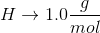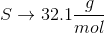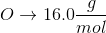Convert the following mass to moles: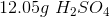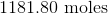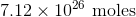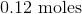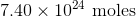Explanation:

To convert grams to moles, use the molecular weight of the molecule. This is found by adding the mass of each atom.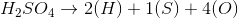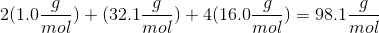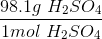Now we can use the molecular mass to convert the given mass in the question to moles. Make sure to align the values so that the units cancel out.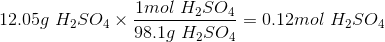### Example Question #2 : Chemistry

How many molecules are in 3.72mol of fluorine?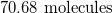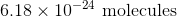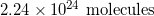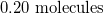Explanation:

Convert from moles to molecules using Avogadro's number: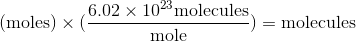Use the moles given in the question to solve.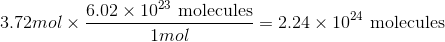### Example Question #2 : Chemistry

Which of the following types of chemical bonds is very strong and involves transfer of an electron between atoms?

Hydrogen bonds

Ionic bonds

Covalent bonds

Disulfide bonds

Ionic bonds

Explanation:

There are two primary bond types: ionic bonds and covalent bonds. Ionic bonds involve the transfer of an electron between atoms, while covalent bonds require atoms to share an electron between them. Ionic bonds are the stronger of the two. Ionic bonds usually form between a metal and a non-metal (for example, NaCl), while covalent bonds usually form between two non-metals (for example, HCl).

Hydrogen bonds are a type of intermolecular force and are similar to a very weak covalent bond. Hydrogen bonds are not actual bonds, and do not chemically link atoms together; they are strictly physical interactions. Disulfide bonds are covalent bonds between sulfur atoms that help form protein structure.

### Example Question #3 : Chemistry

On the periodic table, carbon is atomic numberand has an atomic mass of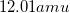. How many sub-atomic particles are found in the nucleus of a carbon atom?Explanation:

The standard sub-atomic particles are protons, neutrons, and electrons. Protons and neutrons are found in the nucleus, while electrons move freely around the nucleus. This question is specifically asking about the number of protons and neutrons in an atom of carbon.

Each proton and each neutron has a mass of approximately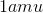. Since the atomic mass of a carbon atom is, we can conclude that there are twelve sub-atomic particles in the nucleus (twelve total protons and neutrons).

The atomic number refers to the number of protons in the nucleus of the atom. Since the atomic number of carbon is six, there are six protons in the nucleus. For this question, however, we need the total of both the protons and neutrons (not just the protons).

### Example Question #3 : Chemistry

A student is asked to make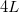of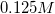NaCl solution. The student is given a stock solution of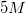NaCl. What volumes of stock NaCl solution and pure water could be used to make the desired final solution?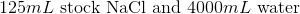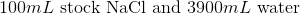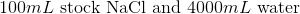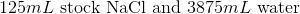Explanation:

Convert liters to mL: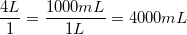This is the TOTAL volume of the solution. Next, determine how much NaCl to add.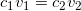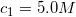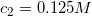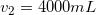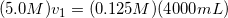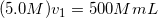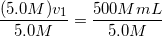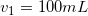Therefore,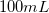of stock NaCl must be added. Subtract this volume from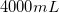to determine the amount of water used in the final solution.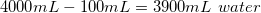### Example Question #6 : Chemistry

6g of sugar is dissolved in 250mL of water. What is the percent concentration of sugar in mass per unit volume?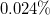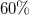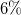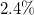Explanation:

Concentration can be measured in a variety of ways. To find the percent mass per unit volume, we must divide the mass of solute by the volume of solvent and multiply by 100%.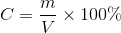We know our mass is 6g and our volume is 250mL. Use these values in the equation to find the concentration.